# NPN BJT transistor: base voltage / emitter current

I don't know how to calculate the voltages at the bases of every transistor shown in the lower row of that picture (~3V (2.9..V), ~2V, ~1.8V). Any help would be appreciated! - I'm currently trying to figure that out...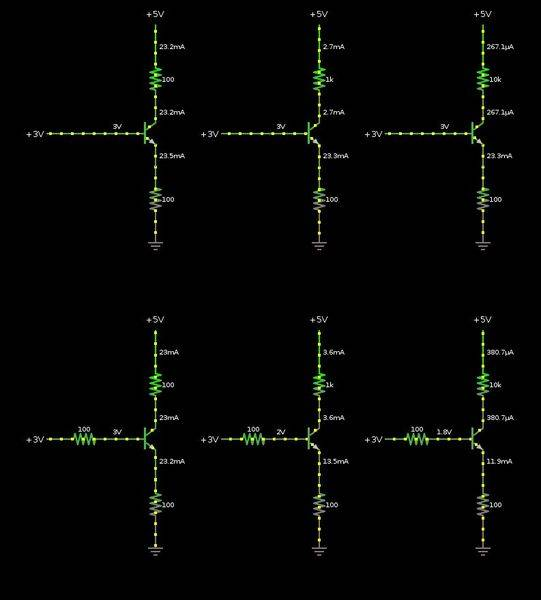The other thing I don't really understand: Why do the transistors in the upper row all have an equal emitter current? Does the the collector-emitter current really only depend on the base current (Ice = Ib * hfe), and not on what's in front of the collector (100 Ohm, 1 kOhm, 10 kOhm)? And why can transistors do this?

(I'm currently trying to learn more about the absolute basics in electronics. - The used simulation tool can be found here. The goal is to understand things like current mirrors and differential amplifiers... but I don't even really get how a simple transistor (BJT) works. Oh well.)

Svein
In the upper row you keep the base voltage constant at +3V. This means that the emitter voltage must be Vbe below +3V or about 2.3V. 2.3V divided by 100Ω (Re) equals 23mA.

In the lower row, the base is not kept at a constant voltage, but at +3V - Rb⋅Ib. Now, Ib depends on Ic and the current gain of the transistor - and Ie = Ic+Ib...

•1rel
The other thing I don't really understand: Why do the transistors in the upper row all have an equal emitter current? Does the the collector-emitter current really only depend on the base current (Ice = Ib * hfe), and not on what's in front of the collector (100 Ohm, 1 kOhm, 10 kOhm)? And why can transistors do this?

Keep in mind that the transistor acts as a voltage-controlled current source (Vbe controls Ic).
This is in accordance with the well-known Shockley-equation: Ic=Is*exp(Vbe/Vt -1) with Vt=temperature voltage (app. 26mV at room temp). The base current Ib is always a small (fixed) portion of the collector current Ib=Ic/B.

That means: It does not matter if the collector resistor Rc is 1k or 10k - the transistor always produces the same current Ic.
However, this applies only as long as the transistor is operated within its active region.
That means: The collector-emitter voltage Vce must not fall below app. 1V.
Otherwise, the transistor is not operated anymore within its "linear" region - and the relationship Ib=Ic/B does not apply.
Therefore: To understand the voltages and currents in the given examples, I recommend to recalculate the various voltages Vce across the transistor. This can be done simply by applying Ohms law for the the collector as well as emitter resistors.

(Note: The above description is the idealized case; in reality, there is a small dependence on Rc resp. Vce because the transistor is, of course, not an IDEAL current source. But this effect is not considered in all transistor models).

Last edited:
•1rel
Thank you for the help!

In the upper row you keep the base voltage constant at +3V. This means that the emitter voltage must be Vbe below +3V or about 2.3V. 2.3V divided by 100Ω (Re) equals 23mA.

Yes, that makes sense!

At the 3 configurations in the top row:
Assuming that the transistor is in its "active region" or even more so in saturation, the collector-emitter voltage drop is small, and the collector-emitter path can be regarded to be a short circuit (right?). So, I can apply Ohm's Law to calculate the currents through the Rc resistors:

(Vcc = 5V and Ve = 5 V - 2.3 V = 2.7 V):
For Rc = 1 kΩ : Ic = ( Vcc - Ve ) / Rc = 2.7 V / 1 kΩ = 2.7 mA
For Rc = 10 kΩ: Ic = ( Vcc - Ve ) / Rc = 2.7 V / 10 kΩ = 270 μA

This seems to coincide with the values shown in the simulation...

But when the transistor is at the edge of becoming active (low base current?), it has a higher resistance on the collector-emitter path and the calcs above don't work anymore.

The simulation shows a collector current Ic of 23.2 mA. So the Rec of the transistor could be calculated like this I guess:

For Rc = 100 Ω and given Ic = 23.2 mA (sim):
Vc = Vcc - Ic⋅Re ) = 5 V - 23.2 mA ⋅ 100 Ω = 2.68 V
(((Rce = Vc / Ic = 2.68 V / 23.2 mA ≈ 116 Ω)))
EDIT: Rce = Vc / Ic - Re = 2.68 V / 23.2 mA - 100 Ω ≈ 16 Ω

How can I really understand this? When I look at the various graphs in datasheets and online I often don't really see how the different values depend on each other, and which value to pick to start the calculation of the values in a given circuit (even as simple as the one above :S). For example, I always thought that the base current is much smaller than the controlled collector/emitter current. But it is not true... It seems like the base/emitter voltage difference is always the same (1 diode drop ≈ 0.6V), and the base voltage somehow controls the SUM of the base-emitter/collector-emitter currents (LvW: yes, a voltage controlled current source. I answer later on that)... So the base current can be in fact much larger than the collector current... hm. I need to work on my model...

In the lower row, the base is not kept at a constant voltage, but at +3V - Rb⋅Ib. Now, Ib depends on Ic and the current gain of the transistor - and Ie = Ic+Ib...

Yes, but I don't really get that yet. I'm trying to figure out how to calculate these values first on paper first, and later hopefully understand the transistor model more intuitively... Probably I build this up on the bread board today.

BTW: The current gain of the simulation transistor model is fixed at 100, which makes it easier to understand the numbers. BTW, here is the simulation file used, now correctly linked (geat simulator by the way with lots of instructive examples)
More later...

Last edited:
1rel, regarding the lower row:
When the transistor is in its active region (lower row, most left circuit), what is the voltage drop across the base resistor caused by the base current (when the current gain is 100)? Is there any severe difference when compared with the most left circuit in the upper row?

•1rel
* The transitor data sheet contains a set of characteristic curves Ic=f(Vce) with parameter Ib or Vbe (in your case: Ib).
* However, due to the rising voltage drops across Rc and Re (for rising current Ic), the remaining voltage for Vce decreases correspondingly.
That means: You cannot have any combination (Ic,Vce) as suggested by the data sheet curves.
That is the meaning of the load line: It shows (crossing points) which combinations (Ic,Vce)are possible for fixed resistors and a fixed supply voltage.

•1rel
1rel, regarding the lower row:
When the transistor is in its active region (lower row, most left circuit), what is the voltage drop across the base resistor caused by the base current (when the current gain is 100)? Is there any severe difference when compared with the most left circuit in the upper row?

Things I can write down after looking at the circuit in the lower row, and convert it to the "linear mode" replacement circuit (see above), I can write down those things about it:

(1) VIN = RB ⋅ IB + VBE + RE ⋅ IE
(2) IC = β ⋅ IB
(3) IE = IC + IB = IB ⋅ (1+β)
(4) VCC = VRC + VCE + VRE
(5) VRC = RC ⋅ IC
(6) VRB = RB ⋅ IB
(7) VRE = RE ⋅ IE
(8) VC = VCC - VRC
(9) VB = VIN - VRB
(10) VE = VB - VBE
(11) VCE = VC - VE
(12) VBE = 0.65 V
(13) β = 100
(14) VIN =3 V
(15) VCC = 5 V

IB = ( VIN - VBE ) / ( RB + RE * ( 1 + β ))
VCE = ( VCC - IB * ( 1 + β ) * RC ) - RC * β * IB
VB = VIN - RB ⋅ IB
IC = β ⋅ IB
VC = VCC - RC * IC
VE = VB - VBE
IE = IC + IB
VCE_check = VC - VE

And get:

RC = 100 Ω, RB = 100 Ω, RE = 100 Ω (lower row, left)
VCE ≈ 0.369 V > 0.2 V
IB ≈ 230 μA > 0.0 A
IC ≈ 23.0 mA
IE ≈ 23.3 mA
VC ≈ 2.70 V
VB ≈ 2.98 V
VE ≈ 2.33 V

That seems to be about equal to the values in the simulation.

But for the other circuits in the lower row, I get negative VCE, so they are in saturation... and I'm still trying to figure out the numbers in the "saturation model" from above...

Last edited:
* The transitor data sheet contains a set of characteristic curves Ic=f(Vce) with parameter Ib or Vbe (in your case: Ib).
* However, due to the rising voltage drops across Rc and Re (for rising current Ic), the remaining voltage for Vce decreases correspondingly.
That means: You cannot have any combination (Ic,Vce) as suggested by the data sheet curves.
That is the meaning of the load line: It shows (crossing points) which combinations (Ic,Vce)are possible for fixed resistors and a fixed supply voltage.

Thank you for the patience :) Yes, I more or less see that, and also tried to play through an example with a resistor and a diode, and a 2 resistors in series. It works -- the crossing points show the actual current at a given voltage drop of over the part plotted in the circuit -- but I will need to work through more examples to really get it, you know... - Please correct me, if I'm wrong about things up there, I'm pretty rusty when it comes to do the number work...

But for the other circuits in the lower row, I get negative VCE, so they are in saturation... and I'm still trying to figure out the numbers in the "saturation model" from above...

I didnt go through all you formulas - nevertheless, one general comment:
In saturation mode (VCE values below threshold) you must not use the beta-values (100). These values are valid for the active amplification mode only.

•1rel
I didnt go through all you formulas - nevertheless, one general comment:
In saturation mode (VCE values below threshold) you must not use the beta-values (100). These values are valid for the active amplification mode only.

Right, I'm taking that crude linear model, and replaced the collector-emitter path by this 0.2 V voltage source (just a constant drop of 0.2 V). However, this is all a bit abstract to me...

In saturation mode (VCE values below threshold) ...

So there are some conditions that say that the NPN BJT is in saturation mode... VCE <= 0.2 V (simplified "linear model" above). And as it seems, it can also be simplified this way: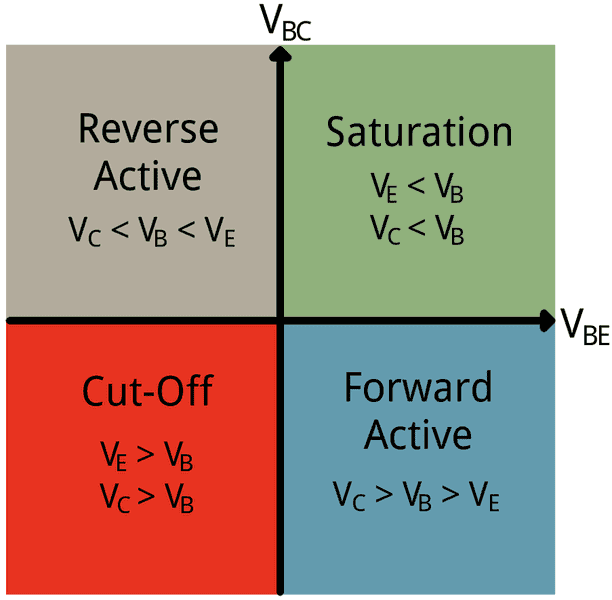According to this chart, the example above should be in saturation mode as well: VB > VC > VE

VC ≈ 2.70 V
VB ≈ 2.98 V
VE ≈ 2.33 V

But I calculated the numbers with the "linear model" replacement circuit from above (VCE ≈ 0.369 V > 0.2 V). So, the transistor's operation point is somewhere at the border between saturation and active region I guess... at least it worked.

When I look into the 2N3904 datasheet... I see those saturation parameters, and it still puzzles me... What's really going on there, how should a simplify this? Is the rule of thumb VB > VC > VE really valid to say, that the transistor is in saturation mode?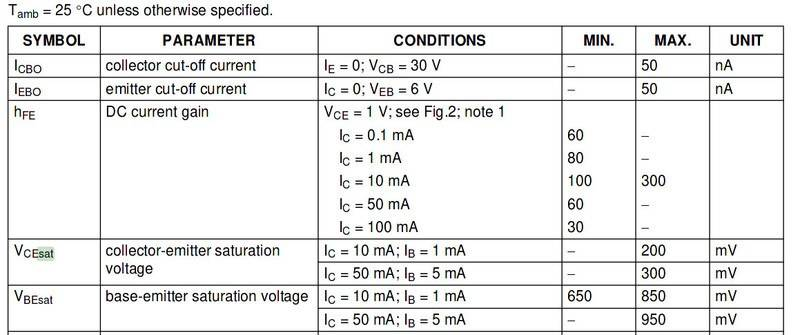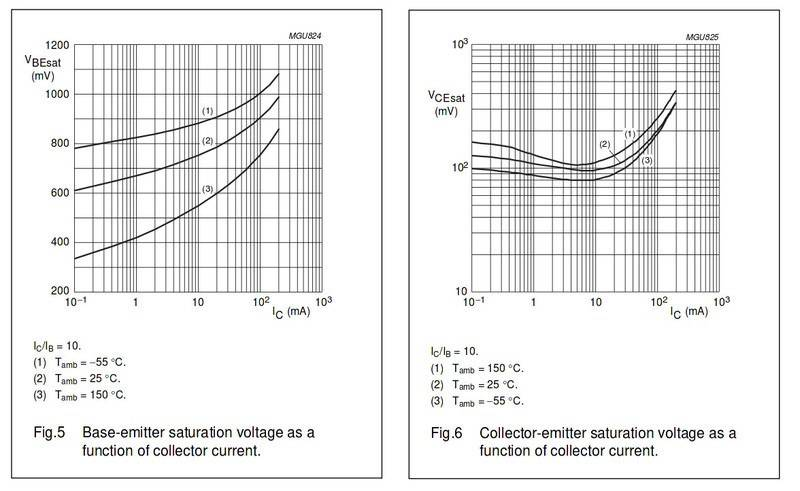It was too much to get the two lower right circuits done on paper, so I played a bit with Sage, and it has a surprisingly nice equation solver in there! The output values differ quite a bit from the values in the simulation. But are they by any means realistic?

That's the program used in sage, maybe it can be opened by this link... (tabs are not right below):

Code:
# global parameters
Vin = 3 # [V]
Vcc = 5 # [V]
beta = 100
Vbe = 0.65 # [V]

def BJT_NPN_Calcs( Rc, Rb, Re ):

print( '-' * 80 )
print( "Rc = %.1f Ohm" % Rc )
print( "Rb = %.1f Ohm" % Rb )
print( "Re = %.1f Ohm" % Re )

Ib = ( Vin - Vbe ) / ( Rb + Re*( 1 + beta ))

if Ib < 0.0:
print( "Cut-off! (Vc=Vcc, Ve=0, Vb=Vin, Ie=Ic=Ib=0)")
else:
Vce = ( Vcc - Ib*( 1 + beta )*Rc ) - Rc*beta*Ib

if Vce >= 0.2:
print( "BJT is in active region" )

Vb = Vin - Rb*Ib
Ic = beta*Ib
Vc = Vcc - Rc*Ic
Ve = Vb - Vbe
Ie = Ic + Ib
Vce_check = Vc - Ve
if( Vce.n( 5 ) != Vce_check.n( 5 ) ):
print( "check 1 failed!" )

else:
print( "BJT is in saturation" )

vars = var('Ic_, Ie_, Ib_')
eq1 = Vcc == Rc*Ic_ + 0.2 + Re*Ie_
eq2 = Vin == Rb*Ib_ + 0.7 + Re*Ie_
eq3 = Ie_ == Ic_ + Ib_
equs = [ eq1, eq2, eq3 ]
sol_n = solve( equs, vars, solution_dict=true )
#sol_x = solve( equs, vars ); sol_x

Ic = sol_n[Ic_]
Ib = sol_n[Ib_]
Ie = sol_n[Ie_]

Vc = Vcc - Rc*Ic
Vb = Vin - Rb*Ib
Ve = Re*Ie

Vc_check = Ve + 0.2
if( Vc.n( 5 ) != Vc_check.n( 5 ) ):
print( "check 1 failed!" )

print( "Ic = %.3f uA" % round( Ic*10^6, 3 ) )
print( "Ib = %.3f uA" % round( Ib*10^6, 3 ) )
print( "Ie = %.3f mA" % round( Ie*10^3, 3 ) )
print( "Vc = %.3f V" % round( Vc, 3 ) )
print( "Vb = %.3f V" % round( Vb, 3 ) )
print( "Ve = %.3f V" % round( Ve, 3 ) )

return

BJT_NPN_Calcs( Rc = 100, Rb = 100, Re = 100 )
BJT_NPN_Calcs( Rc = 1 * 10^3, Rb = 100, Re = 100 )
BJT_NPN_Calcs( Rc = 10 * 10^3, Rb = 100, Re = 100 )

Results:
Code:
--------------------------------------------------------------------------------
Rc = 100.0 Ohm
Rb = 100.0 Ohm
Re = 100.0 Ohm
BJT is in active region
Ic = 23039.216 uA
Ib = 230.392 uA
Ie = 23.270 mA
Vc = 2.696 V
Vb = 2.977 V
Ve = 2.327 V
--------------------------------------------------------------------------------
Rc = 1000.0 Ohm
Rb = 100.0 Ohm
Re = 100.0 Ohm
BJT in saturation
Ic = 3476.190 uA
Ib = 9761.905 uA
Ie = 13.238 mA
Vc = 1.524 V
Vb = 2.024 V
Ve = 1.324 V
--------------------------------------------------------------------------------
Rc = 10000.0 Ohm
Rb = 100.0 Ohm
Re = 100.0 Ohm
BJT in saturation
Ic = 363.184 uA
Ib = 11318.408 uA
Ie = 11.682 mA
Vc = 1.368 V
Vb = 1.868 V
Ve = 1.168 V

After reading a bit more on the topic, I think the configuration above can be seen as a emitter follower.
There are TWO configurations. Only the left circuit is am "emitter follower", if the output is defined at the emitter node.
The second circuit is a common emitter amplfier with negative feedback (also called: emitter degeneration).

Whatever the voltage on the base is doing, the emitter will be doing it as well. The voltage on the emitter VE is always VB - 0.7 V, one diode drop below the base voltage, Vb.
This is a simplified (approximate) view. In reality you must consider a pn-junction like voltage-current characteristic with a base-emitter voltage of approximately 0.6...0.7 V.
As a consequence, the emitter does not EXACTLY follow the base voltage variations.

That can be verified in the two simulation examples above. What's still surprising me, is that the emitter current in both examples is also the same! - It kind of makes sense, because the voltage across the 100 Ω load resistor is always the same (VE = VB - 0.7 V), therefore the current must be VE / RLOAD. However, the current on the left (without a collector resistor) is coming from collector, which is always at +10V. But on the right (with a 10k collector resistor), it is mainly coming from the base of the transistor (IB >> IC)! I still don't get that...
For the most right circuit, the 10v supply does not matter because the voltage drop across the 10k resistor is so large that the collector voltage is only a liitle larger than the emitter voltage. Hence, the charged carriers in the base region are not accelerated by a large collector voltage (forming a large collector current). As a consequence, the only remarkable current is between B and E.

Final comment (recommendation): Do not put too much information into one contribution and do not ask too much - this may keep some forum members from reading all this stuff (information and questions).

Any hints would be appreciated. I have a hard time understanding those BJTs... it would be really nice to have a simple trick to understand them more easily...
There is no "trick" at all. Everything can be explained /described with physical relationships (example npn).:
(1) A base-emitter voltage of app. +0.7V releases electrons from the emitter moving to the base region. Here, they "feel" thath the p-doped region contains only a few electrons - and the electrons start to diffuse into the p-region ("diffusion pressure").
(2) Caused by a collector potential (at least some volts higher than the base potential) there is an electrical field which further accelerates the electrons in the direction to the collector. Thus, they have enough energy to cross the very small base region and reach the collector (forming the collector current). Only a very few move to the base node (forming the base current).
(3) This is a somewhat simplified description of the transistor principle. Note that it is the base-emitter VOLTAGE that determines the amount of electrons leaving the emitter region (emitter current). When 99 % arrive at the collector we have a ratio B=Ic/Ib=99.

Last edited:
•Svein and 1rel
Thank you for the help. This thread kind of blew up a bit, I'm sorry for that... too much info, too many questions.

There are TWO configurations. Only the left circuit is am "emitter follower", if the output is defined at the emitter node.
The second circuit is a common emitter amplfier with negative feedback (also called: emitter degeneration).

Right, the configuration on the right is indeed a common emitter and not a emitter follower anymore, because of that collector resistor. (Emitter degeneration was keyword I didn't know. I'm looking it up... it's about the emitter resistor that makes the configuration more stable.)

The point of comparing those example was about trying to understand the basic BJT behavior. I was wondering why the base current suddenly started rising so much... I kind of get it now.

For the most right circuit, the 10v supply does not matter because the voltage drop across the 10k resistor is so large that the collector voltage is only a liitle larger than the emitter voltage. Hence, the charged carriers in the base region are not accelerated by a large collector voltage (forming a large collector current). As a consequence, the only remarkable current is between B and E.

Exactly! The base current cannot do anything about the collector current, when there's not a high enough voltage to make the charged carriers flow. The transistor is saturated (because of the large collector resistor), and VCE is really small, so the collector-emitter path is basically shorted... both -- base-emitter and base-collector junctions -- are forward biased, and VB ≥ VC > VE. And IC << β * IB. And in this case, the base current is larger than the collector current, because of the large collector resistor.

There's a physical limit for the amount of base current that can flow, compared to collector current, which is smaller than the max collector current I guess?

Looking it up quickly, revealed that the the physical size of the base is not really the limiting factor here *guessing*.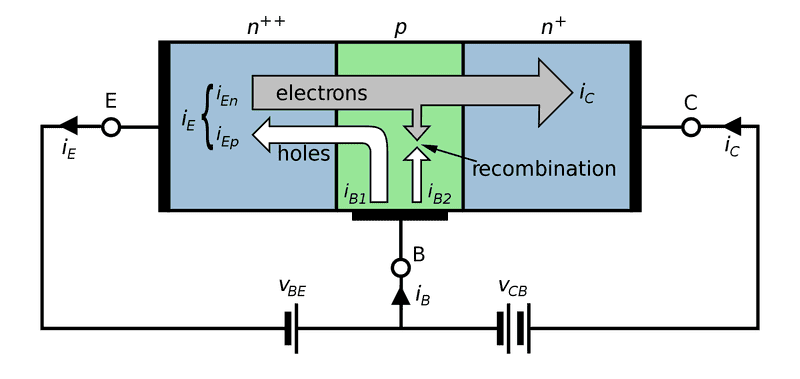Final comment (recommendation): Do not put too much information into one contribution and do not ask too much - this may keep some forum members from reading all this stuff (information and questions).

You're right, sorry for that! The topic is confusing to me, I need to build and measure more basic BJT circuits on the breadboard to understand them better, before asking/answering here in the forum. I was also a bit upset that my understanding of such basic semiconductor behavior and simple volts vs amps graphs etc. is still totally limited. Entering the analog domain now :)

There is no "trick" at all. Everything can be explained /described with physical relationships (example npn).:
(1) A base-emitter voltage of app. +0.7V releases electrons from the emitter moving to the base region. Here, they "feel" thath the p-doped region contains only a few electrons - and the electrons start to diffuse into the p-region ("diffusion pressure").
(2) Caused by a collector potential (at least some volts higher than the base potential) there is an electrical field which further accelerates the electrons in the direction to the collector. Thus, they have enough energy to cross the very small base region and reach the collector (forming the collector current). Only a very few move to the base node (forming the base current).
(3) This is a somewhat simplified description of the transistor principle. Note that it is the base-emitter VOLTAGE that determines the amount of electrons leaving the emitter region (emitter current). When 99 % arrive at the collector we have a ratio B=Ic/Ib=99.

Ah, it's great to look more closely into the physical construction of those semiconductors, instead of those abstract models. The physical charge carriers (electrons/"holes") that move through that tiny n-/p-doped semiconductor...

(1) This behaves about the same like the p-n-junction in a diode. When the 0.6-0.7 V threshold is surpassed, the electrons flow from the n-doped (cathode) silicon to the p-doped (anode).
(2) The electrons that come from the emitter recombine with a few holes coming from the base, but pass through to the collector that is attracting them by a higher voltage (compared to emitter/base - in active mode).
(3) Simplified is good, as long as it helps understanding... β is the ratio of charge carriers/current entering the base versus entering the collector (β = 100: for every electron entering into the base, 100 go to the collector. - The same is also true in reverse, when using conventional current flow... for every charge carrier leaving the base, ...).

@topic voltage controlled current source VS current controlled current source:
A quote there: http://electronics.stackexchange.com/questions/13063/how-do-i-saturate-an-npn-transistor
The truth is that you cannot really separate the base-emitter voltage from the base current, because they are interrelated. So both views are correct. When trying to understand a particular circuit or transistor configuration, I find it is usually best just to pick whichever model makes it easiest to analyze.

Compared to MOSFETs -- which are really voltage controlled (by their gate voltage) -- BJTs always have a base current when they are on, which is again controlled by the voltage present at the base (please correct me if I'm wrong).

Last edited by a moderator:
Compared to MOSFETs -- which are really voltage controlled (by their gate voltage) -- BJTs always have a base current when they are on, which is again controlled by the voltage present at the base (please correct me if I'm wrong).
Yes - BJTs always have a base current IB depending on the existing base-emitter voltage VBE.
More than that , this base current IB is a - nearly - fixed percentage of the collector current IC: IB=IC/B.
As a consequence, some authors (and/or some other contributions) describe the BJT as a "current controlled device" (IC=IB*B).
In some cases and during some design steps such a model-assumption may help to understand what happens - however, the physical truth is:
The BJT is a voltage controlled device following Shockleys equation IC=Is[exp(VBE/Vt) -1].
Many effects and design rules can be explained and verified only based on voltage-control.
But, of course, we must not neglect the base current IB.
There is no doubt - it exists. But it does not "control" IC, it is just a small percentage of IC - thats all.

Hi, let me try to show you how you can use current controlled current source model of the BJT.
And the circuit is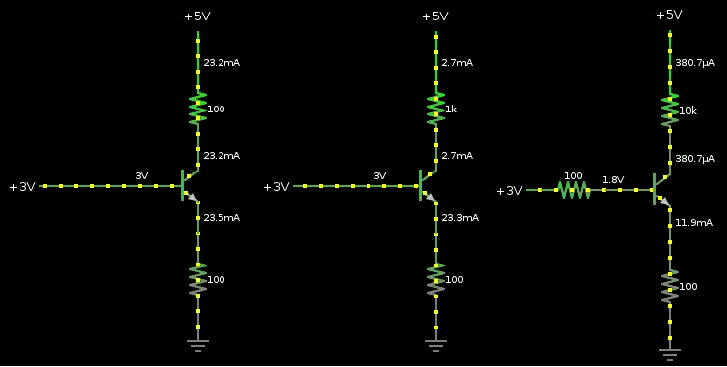At first let we need assume some values. Vbe = 0.6V; β = 100; and Vce(sat) = 0.2V; Vin =3V ; Vcc = 5V;
Now we can apply a KVL for the first circuit
Vin = Vbe + Ie*Re (1)

Ie = (Vin - Vbe)/Re = (3V - 0.6V)/100Ω = 24mA

and Ic = β/(β+1)*Ie = 100/101*24mA = 23.76mA

Ve = Ie*Re = 24mA*100Ω = 2.4V

Vc = Vcc - Ic*Rc = 5V - 23.76mA *100Ω = 2.624V

Vce = Vc - Ve = 2.624V - 2.4V = 0.224V

Transistor is on the edge of a saturation region

Now case (2) Rc = 1kΩ

Ie is still equal to 24mA but now

Vc = Vcc - Ic*Rc = 5V - 23.76mA*1kΩ = -18.76V WOW.
Negative voltage at collector is impossible if your voltage source is 5V the lowest voltage you can get is 0V. Transistor cannot create any voltage. Transistor is not a source of a voltage. Also one of the things that get people super confused is the presumption that the base current is being magnified to form the collector current. But this is not true. What is happening is that the base current is controlling the amount of current that Vcc supplies (sorry LvW I know that you do not like "base current version"). Just like a water tap.
http://obrazki.elektroda.pl/7783290500_1468689739.gif

And this mean (negative voltage on collector) that BJT is in saturation region.
https://www.physicsforums.com/threa...-gain-in-saturation-mode.865392/#post-5432159
And in saturation Ic = Ib*β do not hold any more. The only thing we ca do now is to apply only KCL (Ie = Ib + Ic) and assume Vce(sat) value.
So we have

Ie = 24mA and Ve = 2.4V

Ic = (Vcc - (Vce(sat) + Ve))/Rc = (5V - (0.2+2.4V))/1kΩ = 2.4mA

and Ib = Ie - Ic = 24mA - 2.4mA = 21.6mA

And the last circuit with Rb = 100Ω ; Re = 100Ω; Rc = 10kΩ

Vin = Ib*Rb + Vbe + Ie*Re

Ie = Ib + Ic = Ib + Ib*β = Ib*(β + 1) or Ib = Ie/(β + 1)

Therefore

Vin = Ie/(β + 1)*Rb + Vbe + Ie*Re

Ie = (Vin - Vbe)/( Rb/(β +1 ) + Re ) = (3V - 0.6V)/( 100Ω/101 + 100Ω ) = 23.76mA

Ve = 2.376V

And Vc = Vcc - Ic*Rc = 5V - 100/101*23.76mA * 10kΩ = -230.2V well, what a surprise the BJT is in saturation region.

And because Ic = Ib*β do not hole any more we are force to use Ie = Ib + Ic and Vce(sat) = 0.2V.

Ie = Ib + Ic (1)

Ie = Ve/Re = Ve/100Ω (2)

Ib = (Vin - (Vbe + Ve) )/Rb = ( 3V - (0.6V + Ve) )/100Ω (3)

Ic = (Vcc - (Vce(sat) + Ve )/Rc = (5V - (0.2V + Ve) )/10kΩ (4)

Ve/100Ω = ( 3V - (0.6V + Ve) )/100Ω + (5V - (0.2V + Ve) )/10kΩ ---> solve for Ve

Ve = [ (Vin - Vbe)/Rb + (Vcc-Vce(sat))/Rc ]*(Rg||Rc||Re) = [ (3V - 0.6V)/100Ω + (5V - 0.2V)/10kΩ ] * 100Ω||10kΩ||100Ω = 24.48mA *49.75Ω = 1.217V

so we have
Ie ≈ 1.21V/100Ω = 12mA
Ic ≈(5V - (0.2V+1.21V))/10kΩ = 359μA
Ib ≈ Ie - Ic ≈ 11.6mA

Last edited:
•1rel
Yes - BJTs always have a base current IB depending on the existing base-emitter voltage VBE.
More than that , this base current IB is a - nearly - fixed percentage of the collector current IC: IB=IC/B.
As a consequence, some authors (and/or some other contributions) describe the BJT as a "current controlled device" (IC=IB*B).
In some cases and during some design steps such a model-assumption may help to understand what happens - however, the physical truth is:

With my limited understanding of the subject, I would still agree. The base-emitter voltage VBE, i.e. the actual electrical field inside the transistor between those terminals, is the physical cause of the base current IB, that controls the collector current IC.

A high enough voltage VBE forward biases the P-N junction diode consisting of base and emitter, and let's electrons move from the emitter (N type material in NPN case) over to the base (P type), so that the depletion region (or space charge region!) between those materials is overcome, broken down. Charge carriers (electrons/holes can flow).
Looking at that alone doesn't explain yet why the current can also flow through the emitter-collector path, because the base-emitter P-N junction diode is reverse biased (VB < VC, in active mode). Hm.

Looking it up on Wikipedia revealed some important "details", that I still have difficulties with to really get:
In typical operation, the base–emitter junction is forward biased, which means that the p-doped side of the junction is at a more positive potential than the n-doped side, and the base–collector junction is reverse biased. In an NPN transistor, when positive bias is applied to the base–emitter junction, the equilibrium is disturbed between the thermally generated carriers and the repelling electric field of the n-doped emitter depletion region. This allows thermally excited electrons to inject from the emitter into the base region. These electrons diffuse through the base from the region of high concentration near the emitter towards the region of low concentration near the collector. The electrons in the base are called minority carriers because the base is doped p-type, which makes holes the majority carrier in the base.

To minimize the percentage of carriers that recombine before reaching the collector–base junction, the transistor's base region must be thin enough that carriers can diffuse across it in much less time than the semiconductor's minority carrier lifetime. In particular, the thickness of the base must be much less than the diffusion length of the electrons. The collector–base junction is reverse-biased, and so little electron injection occurs from the collector to the base, but electrons that diffuse through the base towards the collector are swept into the collector by the electric field in the depletion region of the collector–base junction. The thin shared base and asymmetric collector–emitter doping are what differentiates a bipolar transistor from two separate and oppositely biased diodes connected in series.

So the charges that enter the base (where they are always minority carriers - electrons in the NPN case, holes in PNP), recombine with the charges coming from the base terminal, recombine there, and somehow also move over the depletion region to the collector... Although that junction is reverse biased. I don't really get that yet...

@Saturation:
By the way, I've found an interesting configuration called a Schottky Transistor, that prevents the transistor from going into full saturation. - It makes sure, that the voltage at the base only one "schottky drop" (0.2V) higher the the collector, and one diode drop (0.65V) than the emitter. So the transistor never totally saturates...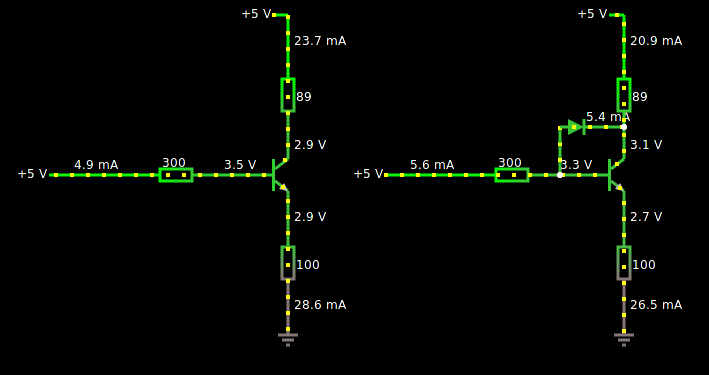(there's no Schottky diode in this simulator)

I thought it's kind of interesting, but doesn't clear the picture up completely yet...

@Transistor as diode:
By shorting the base and the collector of a transistor, it can be transformed into a normal diode. But what's surprising is that the collector/emitter current is always much larger than the base current... I still have the picture of those 2 parallel diodes in my head, oh well... - Come back later to that...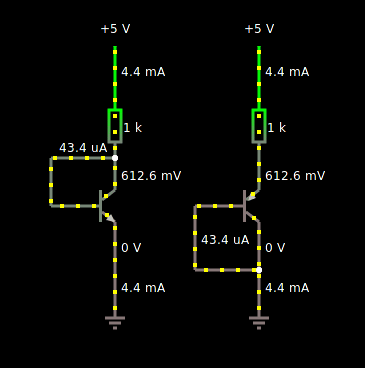The BJT is a voltage controlled device following Shockleys equation IC=Is[exp(VBE/Vt) -1].
Many effects and design rules can be explained and verified only based on voltage-control.
But, of course, we must not neglect the base current IB.
There is no doubt - it exists. But it does not "control" IC, it is just a small percentage of IC - thats all.

You've mentioned that Shockley's equation already, and I've seen it elsewhere too. I'd like to understand it, but have difficulties with more basic things. But yes, it seems to describe the current as a function of voltage... I think it's totally true to say that the BJT is voltage controlled now.

Hi, let me try to show you how you can use current controlled current source model of the BJT.
At first we need assume some values. Vbe = 0.6V; β = 100; and Vce(sat) = 0.2V; Vin =3V ; Vcc = 5V;
Now we can apply a KVL for the first circuit
Vin = Vbe + Ie*Re (1)
Ie = (Vin - Vbe)/Re = (3V - 0.6V)/100Ω = 24mA
Hi Jony130, it took some time to go through your calculations - and I can agree to everything.
However, I have one question:
* Why do you think that you have used the "current controlled current source model of the BJT" ?
For my opinion, you have used all the equations everybody will use (including, of course, Ic=B*Ib).
However, does this mean that you have used a "current-control model"? At which step of the calculation?

* On the other hand, you have used - as an initial assumption - a value of Vbe=0.6 volts. Thats OK.
But are you aware what this means? You need such a voltage to open the transistor - otherwise there will be no current at all!
And if you use 0.7V instead of 0.6V the emitter current will be Ie=(3-0.7)/100=23mA (instead of 24mA).
Where do you see any influence of the base current, which even is not yet calculated ?
Neither the base current nor the factor B were used for finding this value of Ie. Only Vbe !
(Hint for newcomers: Here we see the important influence of an emitter resistor Re: The uncertain assumption of a Vbe voltage - 0.6...0.7V - will have only minor influence on the actual Ie value. Without such a resistor we would face a dramatic and very sensitive dependency between Vbe and Ie, see Shockleys equation).

Also one of the things that get people super confused is the presumption that the base current is being magnified to form the collector current. But this is not true. What is happening is that the base current is controlling the amount of current that Vcc supplies (sorry LvW I know that you do not like "base current version"). Just like a water tap.
http://obrazki.elektroda.pl/7783290500_1468689739.gif
Such a water model looks nice - but it does not reflect the physical working principle of the BJT..
Again: You have calculated Ie without using B or Ib.
How can you say that the base current Ib would control "the amount of current that Vcc supplies" ???

LvW

Last edited:
* Why do you think that you have used the "current controlled current source model of the BJT" ?
For my opinion, you have used all the equations everybody will use (including, of course, Ic=B*Ib).
However, does this mean that you have used a "current-control model"? At which step of the calculation?
Because I didn't use Shockley equation (Ic = Is*(e^(Vbe/Vt) - 1)) anywhere and I treat Vbe as constant voltage source. And for me this is a "current-control model".
And by accident or deliberately in 1rel circuits the emitter current is "controlled" by Vin and Re resistor (emitter current is set by Vin and Re) because Vbe << Vin.
So we don't need to use Ic = Ib*β this time. And in fact this is what we do (or try to do) in real world almost always when we design any circuit using BJT working in linear region.
On the other hand, you have used - as an initial assumption - a value of Vbe=0.6 volts. Thats OK.
But are you aware what this means? You need such a voltage to open the transistor - otherwise there will be no current at all!
And if you use 0.7V instead of 0.6V the emitter current will be Ie=(3-0.7)/100=23mA (instead of 24mA).
Yes, I'm aware what this initial assumption in Vbe means.
Such a water model looks nice - but it does not reflect the physical truth.
Yes, you are right. But you also know that the engineers do not need to know the "truth" to be able to design good and working circuit.
Also this " physics truth" is using very wide range of an abstract models which more or less try to help us understand the mother nature better. The Math is one of those abstract models. I'm bit too philosophical here.

Because I didn't use Shockley equation (Ic = Is*(e^(Vbe/Vt) - 1)) anywhere and I treat Vbe as constant voltage source. And for me this is a "current-control model".
You were not forced to use "explicitely" Shockleys eqation in its exponential form because of emitter degeneration.
Read what I wrote: We have good reason to use always such an emitter resistor - because of the uncertainties connected with transistor parameters (in particular the factor Is).
Using such a resistor, we provide DC negative feedback which makes us - up to a certain degree - independent on the exponential function.
But remember: The values of Vbe=0.6...=0.7 volts are the RESULTS of Shockleys equation for a pn junction!
I think, the fact that you were not forced to use the exponential function means in no way that you have used a current-control model.
Again my question: Where? At which design step?
Yes, you are right. But you also know that the engineers do not need to know the "truth" to be able to design good and working circuit.
Also this " physics truth" is using very wide range of an abstract models which more or less try to help us understand the mother nature better. The Math is one of those abstract models. I'm bit too philosophical here.
Sorry, but I cannot agree. As I have mentioned already, there are many effects and circuits which can be explained (resp. designed) using the voltage control only.
These considerations start already with the question:
* Why do you start your calculations with a FIXED voltage Vbe? Which method do you use to make sure that Vbe is as "stiff" as possible (low resistive voltage division at the base)? This is already a clear proof of voltage control.
* Second esample: Which criteria do we use for selecting a proper quiescent current Ic? We know that a large Ic value will allow larger signal voltage gains. Why? Because the transconductance gm appears in the gain formula; and the transconductance is gm=d(Ic)/d(Vbe). This answers evetything!

Final remark (as I have written not for the first time):
To me, it is most surprising that during design of circuits all people follow the classical design steps for voltage control (of course, that is the only way) - even if they believe they would use a "current-control" model. Up to now, I have no explanation for this phenomenon.

Last edited:
Jony130, because you wrote "I didn't use Shockley equation", I have enclosed a rough scetch to show what really happens.

* There are two exponential curves IE=f(VBE) for two different temperatures or two different factors Is. More than that, there is a "working line" for determining the resulting operational point (qiescent emitter current IE).
* For a finite value of RE, the resulting current IE moves from "1" to "2" (in our case, from 23 to 24 mA for VBE=0.7 resp 0.6 volts). Just a relatively small change (uncertainty).
* For RE=0 the working line is a vertical line, which would result in a much larger emitter current (point "3") when the temperature or the factor Is would have a larger value. In this case and for a desired value for IE we must know the exact temperature as well as the position of the exponenetial curve, which is defined by the factor Is. As you know, this factor is not known (resp. with very large tolerances only).
* The drawing clearly shows - if RE has a finite value - why it matters not too much if we start calculations with 0.6 or 0.7 volts. The resulting uncertainty in IE is acceptable and cannot be avoided!
But these values result from Shockleys exponential function!

#### Attachments

• Operating points.pdf
311.6 KB · Views: 332
But how can a fixed Vbe voltage "control" current? For DC operating point analysis (post #17 ) I used this simplified circuit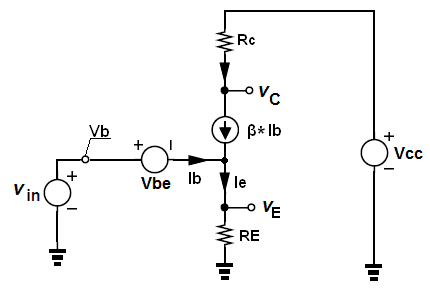And as you can see this model use a CCCS as a representation of a BJT plus the fixed Vbe voltage. And because the property of a circuit topology given by TS is like this. We can clearly seen that Ie = (Vin - Vbe)/Re. And I use this (mental) model (CCCS + fixed Vbe) to do the DC operating point analysis for almost all the circuit. I almost never use Shockley's equation, but yes, there are some rare situation when Shockley's equation is needed.
Also I do not see how this Vbe = 0.6...0.7 volts are the results of Shockley's equation? Vbe value is strongly dependent on junction area (power BJT will have a smaller Vbe for a given current) and some other factors. And this common values for Vbe are just a simple the rule of thumb. But I think that we are way off-topic now.
Also do not get me wrong I know that BJT is a VCCS device.

But how can a fixed Vbe voltage "control" current? (post #17 )
Look at the first line of your calculation in post#17:
Ie = (Vin - Vbe)/Re = (3V - 0.6V)/100Ω = 24mA
I see two voltages (Vin and Vbe) which determine resp. control the emitter current. This equation is nothing else than the "working line" as shown in my pdf graph.
When we have the emitter current, we simply can derive the two other currents (Ib and Ic) using the known relationships Ie=Ic+Ib and Ic=beta*Ib.
Thats what your model does using a current source which establishes the multiplication (beta*Ib). But this does not mean that Ic would be "controlled" by Ib.
You have started with Ie=f(Vbe). Hence, Ie depends on Vbe.

I almost never use Shockley's equation, but yes, there are some rare situation when Shockley's equation is needed.
Also I do not see how this Vbe = 0.6...0.7 volts are the results of Shockley's equation? Vbe value is strongly dependent on junction area (power BJT will have a smaller Vbe for a given current) and some other factors. And this common values for Vbe are just a simple the rule of thumb.
Each rule of thumb is derived either from (a) theoretical considerations or (b) practical experiments.
Here we speak about the classical exponential expression which describes the current-voltage relation for any pn junction (Si or Ge).
This function was first introduced by W. Shockley and this function is the source of the simplified view which allows us to assume an estimated value for Vbe (0.6...0.7 volts).

(*Catching up, answering to previous posts here...*)

Jony130: That was of great help understanding the initial example! Thanks a lot for the examples, those will come in handy in many situations!

Jony130 said:
And because Ic = Ib*β do not hole any more we are force to use Ie = Ib + Ic and Vce(sat) = 0.2V.

Ie = Ib + Ic (1)

Ie = Ve/Re = Ve/100Ω (2)

Ib = (Vin - (Vbe + Ve) )/Rb = ( 3V - (0.6V + Ve) )/100Ω (3)

Ic = (Vcc - (Vce(sat) + Ve )/Rc = (5V - (0.2V + Ve) )/10kΩ (4)

Ve/100Ω = ( 3V - (0.6V + Ve) )/100Ω + (5V - (0.2V + Ve) )/10kΩ ---> solve for Ve

Ve = [ (Vin - Vbe)/Rb + (Vcc-Vce(sat))/Rc ]*(Rg||Rc||Re) = [ (3V - 0.6V)/100Ω + (5V - 0.2V)/10kΩ ] * 100Ω||10kΩ||100Ω = 24.48mA *49.75Ω = 1.217V

so we have
Ie ≈ 1.21V/100Ω = 12mA
Ic ≈(5V - (0.2V+1.21V))/10kΩ = 359μA
Ib ≈ Ie - Ic ≈ 11.6mA

I was able to follow until the last example (RC = 10 kΩ).

That's where I got stuck...

100Ω||10kΩ||100Ω

That means those 3 resistors in parallel, right? Rtot = 1 / ( 2/100Ω + 1/10000Ω ) ≈ 49.8 Ω

In sage gives almost the same result:
Code:
e = 100
Rb = 100
Rc = 10000
Vin = 3
Vcc = 5
Vbe = 0.6
Vcesat = 0.2

vars = var('Ic_, Ie_, Ib_, Ve_')
eq1 = Ie_ == Ib_+ Ic_
eq2 = Ie_ == Ve_  / Re
eq3 = Ib_ == ( Vin - ( Vbe + Ve_ ) ) / Rb
eq4 = Ic_ == ( Vcc - ( Vcesat + Ve_ ) ) / Rc
equs = [ eq1, eq2, eq3, eq4 ]
sol_n = solve( equs, vars, solution_dict=true )
#sol_x = solve( equs, vars ); sol_x

sol_n[Ie_].n(50)
sol_n[Ic_].n(50)
sol_n[Ib_].n(50)

[[Ic_ == (3/8375), Ie_ == (102/8375), Ib_ == (99/8375), Ve_ == (408/335)]]
0.012179104477612
0.00035820895522388
0.011820895522388

I want to come back solving that by hand later on...

Jony130 said:
https://www.physicsforums.com/threa...-gain-in-saturation-mode.865392/#post-5432159 [Broken]

That's interesting! I never know how to read those parameters in the datasheet, and what resistor to choose to make the transistor really work as a switch (i.e. in its saturated mode).

Ib > (Vcc/Rc)/β will saturate our BJT.

Overdrive of 10 is fine?

LvW: @graph

Aside from the CCVS-VS-CCCS discussion (which I cannot really contribute to), the operating points graph in the PDF, showing IE as a function of VBE of the transistor's base-emitter P-N junction. It looks indeed like a normal I-V graph of a diode! And the linear IE work-/loadline describes the emitter current through the emitter resistor depending on VBE (?)... actual current can be determined by crossing the two functions, each crossing shows the actual base current (non-linear), at a certain base-emitter voltage... - All new to me, those load line concepts, but they seems to be really useful for understanding/visualizing the actual behavior, to build a mental model that helps analyzing/build circuits more intuitively.

@physical model:

I've found a really good explanation of the physics of the BJT there: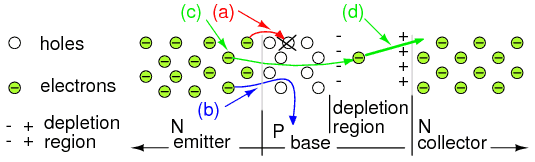The answer to the earlier question about the conducting reverse-biased base-collector junction seems to be, that the P-N junction in the NPN BJT in active mode is conducting, because the electrons actually get into the depletion region of the P-N junction between base and collector. The base is really thin! So most of the electrons (minority carriers in the base) flow from the P material (lots of holes) of the base to the N-doped silicon of the large collector, because there's an electric potential/field in the depletion region that makes the surplus of electrons in the base jump over to the N-doped collector, where they can flow out through the collector terminal. How many per second? That depends on VBE and VCE. IB only makes sure that the depletion layer between base and emitter is "broken down", i.e. the junction is forward biased, thus "weakend", so that current can pass (0.6-0.7 V). How much electron current can flow from the emitter to the base (NPN) depends on VBE, like in a diode (?) (edit: not sure about this...).

Last edited by a moderator:
LvW: @graph
Aside from the CCVS-VS-CCCS discussion (which I cannot really contribute to), the operating points graph in the PDF, showing IE as a function of VBE of the transistor's base-emitter P-N junction. It looks indeed like a normal I-V graph of a diode! And the linear IE work-/loadline describes the emitter current through the emitter resistor depending on VBE (?)... actual current can be determined by crossing the two functions, each crossing shows the actual base current (non-linear), at a certain base-emitter voltage... - All new to me, those load line concepts, but they seems to be really useful for understanding/visualizing the actual behavior, to build a mental model that helps analyzing/build circuits more intuitively.

Just a small correction: Emitter current (instead of base current).

That's where I got stuck...
Why? And where ?

That means those 3 resistors in parallel, right?
Yes, R1||R2 ----> parallel-----> 1/(1/R1 +1/R2)

And the Ve equation for case 3

$$V_E\ =\ \left(\frac{Vin-Vbe}{R_B}+ \frac{Vcc - Vce(sat)}{R_C}\right)* R_B||R_E||R_C$$
Overdrive of 10 is fine?
Most of the time yes, but as everything in electronics it's depend on a given circuit/application.
How much electron current can flow from the emitter to the base (NPN) depends on VBE, like in a diode
But in BJT we want high current gain so, the base current should be as small as possible. And Vbe voltage "sets" how much electrons is injected from emitter to base. But because the base is very thin the small number of electrons will recombine in the base. Also because the collector voltage is much larger then vbe this E field will sweeps those electrons into the collector (drift current due to large electric field (Vcc>>Vbe) ). So that almost all electrons that whose inject into emitter will reach collector terminal.

Last edited by a moderator:
•1rel